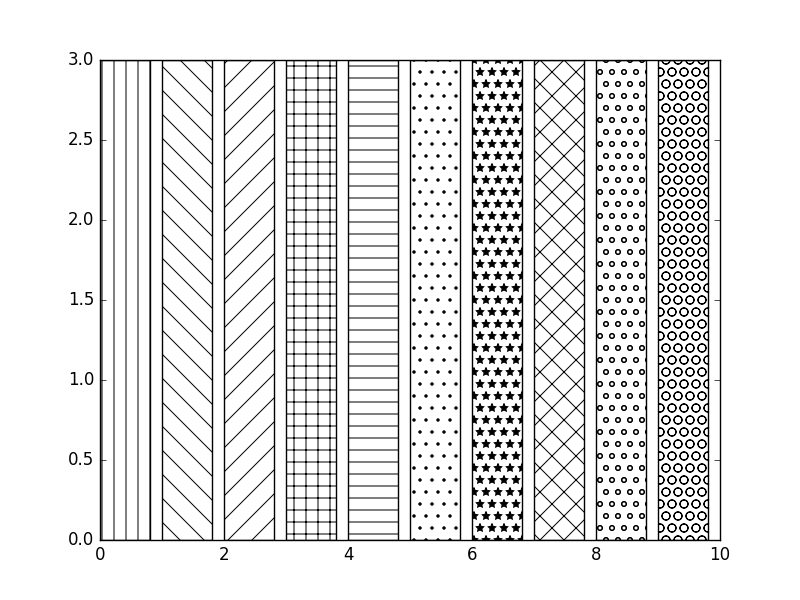﻿ Matplotlib Bar Chart: Display textures to bars and wedges - w3resource# Matplotlib Bar Chart: Display textures to bars and wedges

## Matplotlib Bar Chart: Exercise-17 with Solution

Write a Python program to add textures (black and white) to bars and wedges.

Sample Solution:

Python Code:

``````import matplotlib.pyplot as plt
fig = plt.figure()
patterns = [ "|" , "\\" , "/" , "+" , "-", ".", "*","x", "o", "O" ]

for i in range(len(patterns)):
ax.bar(i, 3, color='white', edgecolor='black', hatch=patterns[i])

plt.show()
```
```

Sample Output:Python Code Editor:

Have another way to solve this solution? Contribute your code (and comments) through Disqus.

What is the difficulty level of this exercise?

﻿